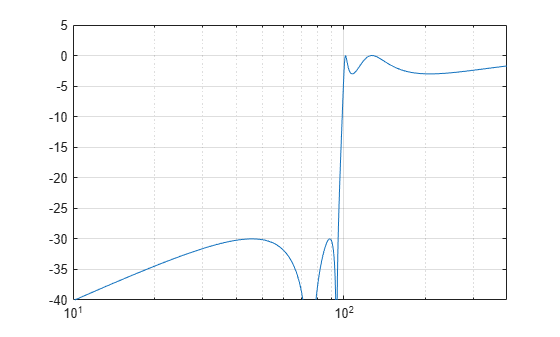# lp2hp

Transform lowpass analog filters to highpass

## Syntax

``[bt,at] = lp2hp(b,a,Wo)``
``[At,Bt,Ct,Dt] = lp2hp(A,B,C,D,Wo)``

## Description

example

````[bt,at] = lp2hp(b,a,Wo)` transforms an analog lowpass filter prototype given by polynomial coefficients (specified by row vectors `b` and `a`) into a highpass analog filter with cutoff angular frequency `Wo`. The input system must be an analog filter prototype.```
````[At,Bt,Ct,Dt] = lp2hp(A,B,C,D,Wo)` converts the continuous-time state-space lowpass filter prototype (specified by matrices `A`, `B`, `C`, and `D`) to a highpass analog filter with cutoff angular frequency `Wo`. The input system must be an analog filter prototype.```

## Examples

collapse all

Design a 5th-order highpass elliptic filter with a cutoff frequency of 100 Hz, 3 dB of passband ripple, and 30 dB of stopband attenuation

Design the prototype. Convert the zero-pole-gain output to a transfer function.

```f = 100; [ze,pe,ke] = ellipap(5,3,30); [be,ae] = zp2tf(ze,pe,ke);```

Transform the prototype to a highpass filter. Specify the cutoff frequency in rad/s.

`[bh,ah] = lp2hp(be,ae,2*pi*f);`

Compute and plot the frequency response of the filter. Divide the normalized frequency by $2\pi$ so the x-axis of the plot is in Hz.

```[hh,wh] = freqs(bh,ah,4096); semilogx(wh/2/pi,mag2db(abs(hh))) axis([10 400 -40 5]) grid```## Input Arguments

collapse all

Prototype numerator and denominator coefficients, specified as row vectors. `b` and `a` specify the coefficients of the numerator and denominator of the prototype in descending powers of s:

`$\frac{B\left(s\right)}{A\left(s\right)}=\frac{b\left(1\right){s}^{n}+\cdots +b\left(n\right)s+b\left(n+1\right)}{a\left(1\right){s}^{m}+\cdots +a\left(m\right)s+a\left(m+1\right)}$`

Data Types: `single` | `double`

Prototype state-space representation, specified as matrices. The state-space matrices relate the state vector x, the input u, and the output y through

`$\begin{array}{l}\stackrel{˙}{x}=Ax+Bu\\ y=Cx+Du\end{array}$`

Data Types: `single` | `double`

Cutoff angular frequency, specified as a scalar. Express the cutoff angular frequency in rad/s.

Data Types: `single` | `double`

## Output Arguments

collapse all

Transformed numerator and denominator coefficients, returned as row vectors.

Transformed state-space representation, returned as matrices.

## Algorithms

`lp2hp` transforms analog lowpass filter prototypes with a cutoff angular frequency of 1 rad/s into highpass filters with a desired cutoff angular frequency. The transformation is one step in the digital filter design process for the `butter`, `cheby1`, `cheby2`, and `ellip` functions.

`lp2hp` is a highly accurate state-space formulation of the classic analog filter frequency transformation. If a highpass filter is to have a cutoff angular frequency ω0, the standard s-domain transformation is

`$s=\frac{{\omega }_{0}}{p}.$`

The state-space version of this transformation is:

```At = Wo*inv(A); Bt = -Wo*(A\B); Ct = C/A; Dt = D - C/A*B; ```

See `lp2bp` for a derivation of the bandpass version of this transformation.

## Version History

Introduced before R2006a